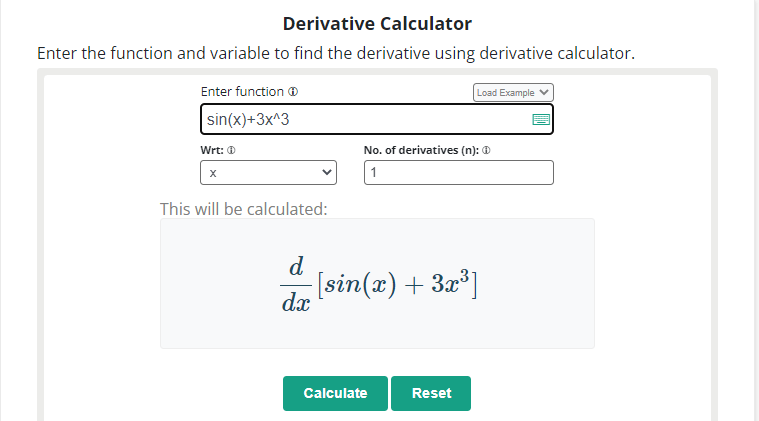Management Notes

# Management Notes

Reference Notes for Management

# Math Homework Help: The Best Websites for Students

The difficulty of math homework can vary greatly depending on the individual student’s understanding and background in the subject, as well as the specific assignment or problem.

Some students may find certain topics or concepts more difficult than others, while others may find the entire subject challenging. Additionally, the difficulty of math homework can be influenced by factors such as the teaching method used, the textbook or materials used, and the student’s study habits and approach to learning.

If you are struggling with your math homework, there are several things you can do to help improve your understanding and make the material easier to grasp. These include:

• Reviewing class notes and past assignments
• Practicing problems and working through sample problems
• Asking your teacher or a tutor for help and clarification on difficult concepts
• Using online resources such as calculators, videos, tutorials, and interactive exercises to supplement your learning
• Breaking down problems into smaller, manageable parts and approaching them step-by-step
• Consistently reviewing and practicing math concepts on a regular basis

It’s important to note that math is a subject that builds on itself, so it is important to keep up with the material and not to fall behind Math can be a challenging subject for many students, but fortunately, there are online resources available to help. Here are some of the best websites for math homework help:

Khan academy offers a wide range of math tutorials, from basic arithmetic to advanced calculus. The tutorials are presented in a clear and easy-to-understand format, and there are also practice problems and quizzes to test your understanding.This website can help you to understand any topic of mathematics from the beginning. After taking assistance from the online tutorials of this website, you would be able to solve any mathematics homework and assignment.

For example, if you want to take the basic concepts of summation notation. Search for summation notation and take the tutorials to understand the basics of this topic.

## 2.  Allmath.com

Allmath is a calculator website that offers 400+ calculators for mathematics, physics, chemistry, statistics, and daily life conversions.This website is a helpful resource for students for solving the numerical problems of their homework and assignments. Students can search for their desired tool and can easily evaluate their problems by entering the inputs.

Tools offered by Allmath will provide the step by step solutions to the given problems. For example, if you want to evaluate the differential of sin2(x) + x2 with respect to “x”. Search for the derivative calculator and enter the input in the required input field & select the variable.Simply press the calculate button to get the result.Allmath offered various calculators for algebra, trigonometry, calculus, and geometry. Such as

• Limit Calculator
• Slope Intercept Form Calculator
• Two’s Complement Calculator
• Proportion Calculator
• And so on.

## 3.  Math.com

This website offers a variety of math resources, including lessons, games, and worksheets. It covers a wide range of math topics, from basic arithmetic to advanced calculus.There are various online tools available on this website for helping the student to learn mathematics and for solving homework questions. Students can take tutorials on different math topics and solve practice problems to test themselves.

Tools offered by math.com are:

These are some of the best websites for math homework help. Each of them offers a unique set of features and resources to help you understand and solve math problems.